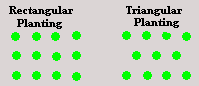Plants Needed for Planting a Right-Angled Triangle Shaped Area (Metric)Calculate the amount of plants needed to plant a right-angled triangle area.

This calculator calculates the area to be planted in square metres and the required amount of plants for triangular and square pattern planting.

Unit of measurements used for this calculator is Metric, metres and centimetres

Length A: M
Length B: M
Plant Spacing: cm

Results:

 Square Metres of Area: M² Number of Plants Needed: Using a Square Pattern Style Planting Number of Plants Needed: Using a Triangular Pattern Style Planting